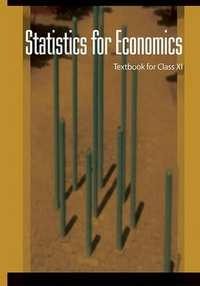# NCERT solutions for Economics - Statistics for Economics Class 11 chapter 7 - Correlation [Latest edition]

#### Chapters## Chapter 7: Correlation

Exercise
Exercise [Pages 105 - 106]

### NCERT solutions for Economics - Statistics for Economics Class 11 Chapter 7 CorrelationExercise [Pages 105 - 106]

Exercise | Q 1. | Page 105

The unit of the correlation coefficient between height in feet and weight in kgs is ______

• kg/feet

• percentage

• non-existent

Exercise | Q 2. | Page 105

The range of simple correlation coefficient is ______

• 0 to infinity

• minus one to plus one

• minus infinity to infinity

Exercise | Q 3. | Page 105

If rxy is positive the relation between X and Y is of the type ______

• When Y increases X increases

• When Y decreases X increases

• When Y increases X does not change

Exercise | Q 4. | Page 105

If rxy = 0 the variable X and Y are ______

• linearly related

• not linearly related

• independent

Exercise | Q 5. | Page 105

Of the following three measures which can measure any type of relationship.

• Karl Pearson’s coefficient of correlation

• Spearman’s rank correlation

• Scatter diagram

Exercise | Q 6. | Page 105

If precisely measured data are available the simple correlation coefficient is ______

• more accurate than the rank correlation coefficient

• less accurate than the rank correlation coefficient

• as accurate as the rank correlation coefficient

Exercise | Q 7. | Page 105

Why is r preferred to covariance as a measure of association?

Exercise | Q 8. | Page 105

Can r lie outside the –1 and 1 range depending on the type of data?

Exercise | Q 9. | Page 105

Does correlation imply causation?

Exercise | Q 10. | Page 105

When is rank correlation more precise than simple correlation coefficient?

Exercise | Q 11. | Page 105

Does zero correlation mean independence?

Exercise | Q 12. | Page 105

Can a simple correlation coefficient measure any type of relationship?

Exercise | Q 13. | Page 105

Collect the price of five vegetables from your local market every day for a week. Calculate their correlation coefficients. Interpret the result.

Exercise | Q 14. | Page 105

Measure the height of your classmates. Ask them the height of their benchmate. Calculate the correlation coefficient of these two variables. Interpret the result.

Exercise | Q 15. | Page 106

List some variables where accurate measurement is difficult.

Exercise | Q 16. | Page 106

Interpret the values of r as 1, –1, and 0.

Exercise | Q 17. | Page 106

Why does the rank correlation coefficient differ from the Pearsonian correlation coefficient?

Exercise | Q 18. | Page 106

Calculate the correlation coefficient between the heights of fathers in inches (X) and their sons (Y)

 X 65 66 57 67 68 69 70 72 Y 67 56 65 68 72 72 69 71
Exercise | Q 19. | Page 106

Calculate the correlation coefficient between X and Y and comment on their relationship:

 X -3 -2 -1 1 2 3 Y 9 4 1 1 4 9
Exercise | Q 20. | Page 106

Calculate the correlation coefficient between X and Y and comment on their relationship.

 X 1 3 4 5 7 8 Y 2 6 8 10 14 16

## Chapter 7: Correlation

Exercise## NCERT solutions for Economics - Statistics for Economics Class 11 chapter 7 - Correlation

NCERT solutions for Economics - Statistics for Economics Class 11 chapter 7 (Correlation) include all questions with solution and detail explanation. This will clear students doubts about any question and improve application skills while preparing for board exams. The detailed, step-by-step solutions will help you understand the concepts better and clear your confusions, if any. Shaalaa.com has the CBSE Economics - Statistics for Economics Class 11 solutions in a manner that help students grasp basic concepts better and faster.

Further, we at Shaalaa.com provide such solutions so that students can prepare for written exams. NCERT textbook solutions can be a core help for self-study and acts as a perfect self-help guidance for students.

Concepts covered in Economics - Statistics for Economics Class 11 chapter 7 Correlation are Lorenz Curve, Measures of Central Tendency - Mean, Measures of Central Tendency - Median, Measures of Central Tendency - Mode, Measures of Central Tendency - Central Value Or Representative Value, Measures of Central Tendency - Series, Measures of Central Tendency - Partition Value, Measures of Central Tendency - Quartile, Measures of Central Tendency - Deciles, Measures of Central Tendency - Percentile, Measures of Dispersion - Absolute Dispersion, Measures of Dispersion - Relative Dispersion, Lorenz Curve, Correlation Meaning, Properties of Correlation,, Types of Correlation, Scatter Diagram of Correlation, Measures of Correlation - Karl Pearson'S Method (Two Variables Ungrouped Data), Measures of Correlation - Spearman's Rank Correlation, Meaning of Index Numbers, Uses of Index Numbers, Types of Index Number - Wholesale Price Index, Types of Index Number - Consumer Price Index, Types of Index Number - Index of Industrial Production, Construction of Price Index, Cost of Living Index Number, Inflation and Index Numbers, Method to Measure - Simple Aggregate Method, Method to Measure - Simple Average Price Relative Method, Method to Measure - Laspeyer Method, Method to Measure - Paasche's Index Numer, Method to Measure - Fisher's Method, Correlation Meaning, Types of Relationship, Techniques for Measuring Correlation, Correlation - Conclusion.

Using NCERT Class 11 solutions Correlation exercise by students are an easy way to prepare for the exams, as they involve solutions arranged chapter-wise also page wise. The questions involved in NCERT Solutions are important questions that can be asked in the final exam. Maximum students of CBSE Class 11 prefer NCERT Textbook Solutions to score more in exam.

Get the free view of chapter 7 Correlation Class 11 extra questions for Economics - Statistics for Economics Class 11 and can use Shaalaa.com to keep it handy for your exam preparation